# Class 8 NCERT Solutions – Chapter 4 Practical Geometry – Exercise 4.1

• Last Updated : 04 May, 2021

### (i) Quadrilateral ABCD      AB = 4.5 cm, BC = 5.5 cm, CD = 4 cm, AD = 6 cm, AC = 7 cm

Procedure:

Given AB = 4.5 cm, BC = 5.5 cm, CD = 4 cm, AD = 6 cm, AC = 7 cm
We have to construct a quadrilateral ABCD

Attention reader! All those who say programming isn't for kids, just haven't met the right mentors yet. Join the  Demo Class for First Step to Coding Coursespecifically designed for students of class 8 to 12.

The students will get to learn more about the world of programming in these free classes which will definitely help them in making a wise career choice in the future.

• Draw a rough sketch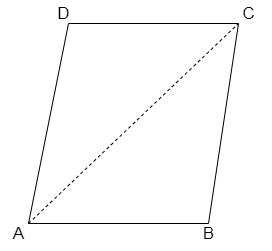• Draw AB = 4.5 cm.
• Taking B as centre, draw an arc of radius 5.5 cm.
• Now taking A as the centre, draw an arc of radius 7 cm intersecting with the arc made previously with centre B. Mark the intersection point as point C. Join AC and BC, successively.
• Taking A as the centre again, draw an arc of radius 6 cm.
• Taking D as the centre (which was marked previously), draw an arc of radius 4 cm, now intersect both the arcs, one of radius 6 cm made from point A and the other made from point C, and mark the intersection point as D (update the rough point).
• Now join AD and CD.
• Hence we have the required quadrilateral ABCD.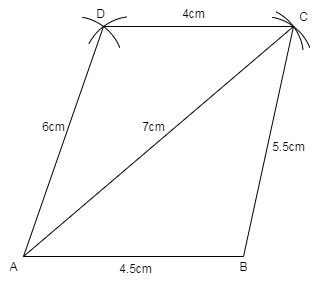### (ii) Quadrilateral JUMP       JU = 3.5 cm, UM = 4 cm, MP = 5 cm, PJ = 4.5 cm, PU = 6.5 cm

Procedure:

Given JU = 3.5 cm, UM = 4 cm, MP = 5 cm, PJ = 4.5 cm, PU = 6.5 cm
We have to construct a quadrilateral JUMP

• Draw JU = 3.5 cm.
• Taking J as centre draw an arc of radius 4.5 cm and taking U as centre draw an arc of 6.5 cm, such that both the arcs should intersect at point P. Join PJ and PU respectively.
• Similarly, taking P and U as centre draw arcs of 5 cm and 4 cm respectively, intersect both the arcs at M.
• Join MP and UM.
• Hence we have the required quadrilateral.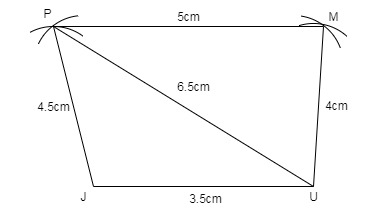### (iii) Parallelogram MORE        OR = 6 cm, RE = 4.5 cm, EO = 7.5 cm

Procedure:

Given OR = 6 cm, RE = 4.5 cm, EO = 7.5 cm
We have to construct a parallelogram MORE (Opposite sides are equal in a parallelogram).

• Draw an arc of 4.5 cm taking R as the centre.
• Draw an arc of 7.5 cm taking O as the centre, such that it intersects the previous arc.
• Join both the arcs at point E. Join EO and RE
• Taking E as centre draw an arc of 6 cm.
• Taking O as centre draw an arc of 4.5 cm.
• Intersect both the arcs at M. Join EM and OM.
• Therefore we have the required parallelogram MORE.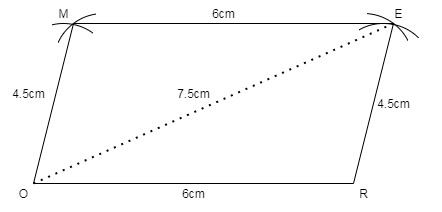### (iv) Rhombus BEST        BE = 4.5 cm, ET = 6 cm

Procedure:

Given BE = 4.5 cm, ET = 6 cm
We have to construct a Rhombus BEST (all sides of a rhombus are equal)

• Draw a triangle BET,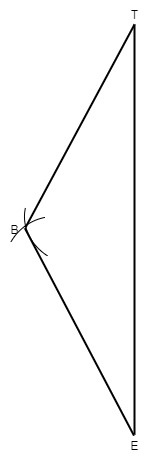• Draw ET = 6 cm.
• Draw an arc of 4.5 cm from both the points E and T respectively, join BE and BT.
• Similarly, taking E and T as centre draw arcs of 4.5 cm and intersect at S as shown below,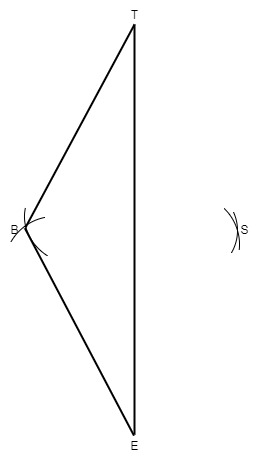• Join SE and ST.
• Join Diagonal BE. Measure it will be 4.5 cm.
• Therefore, we have the required Rhombus BEST.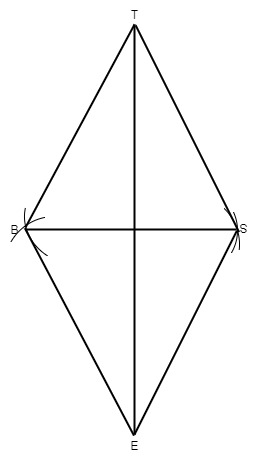My Personal Notes arrow_drop_up